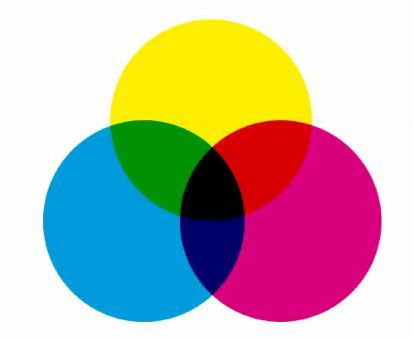CMYK Color | Html Colors

# CMYK Color

CMYK Color, is a subtractive model and is used in printing from pigments of three basic colors: C - cyan, M - magenta and Y - yellow. The K comes from the black, since the combination of the three previous ones produces a black little pure, for that reason a pure black pigment is added to the model. Unlike in RGB, where black is the absence of light, in CMYK white is represented here as the absence of pigments.
The intermediate colors are produced from the mixture in different proportions of the base pigments.
There is a relationship between the RGB and CMYK models, since with the mixture of each of the basic colors of one model, we obtain the primary colors of the other.
In RGB (red, green, blue):
Red and green in equal proportions: we get yellow - Y from CMYK
Red and blue in equal proportions: we get magenta - M
Green and blue in equal proportions: we get the cyan - C
In CMYK (cyan, magenta, yellow):
Cyan and magenta in equal proportion: we get the blue
Cyan and yellow in equal proportion: we get the green
Magenta and yellow in equal proportion: we get the red